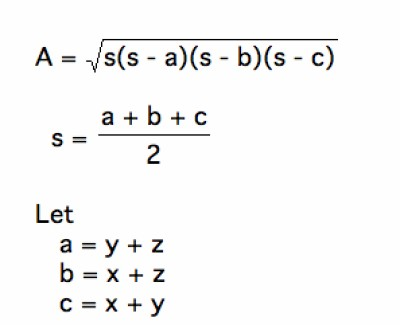List of public pages created with Protopage

# Home

## Plain sticky notes

I am a year 10 student, 15 years old. I enjoy all sports but particularly football. I play for a football team and I'm part of an athletics club.

# Simple and Compound interest

## Photos

### Simple and compound interesrt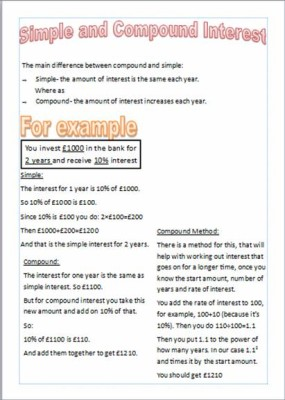# Graphs

## Plain sticky notes

### Straight line graphs

Straight line graphs show a a line slanting to the right or left. For example, with Y=3X+1 you would end up with a graph like 'Figure 1'.

Quadratic graphs create a single curve in the line. (Figure 2)

### Cubic graphs

Cubic Graphs create a double curve, one to the right and the other to the left. (Figure 3)

## Photos

Figure 2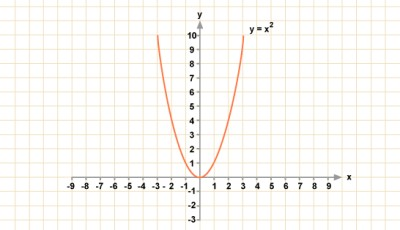### Cubic graph

Figure 3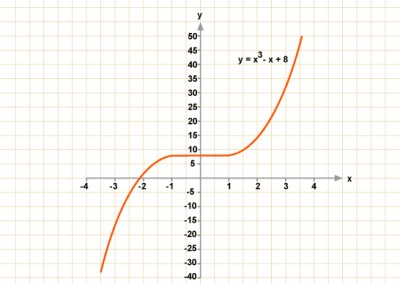### Straight graph

Figure 1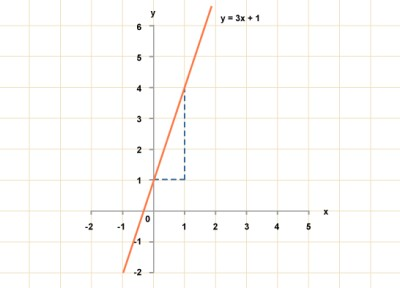# Histograms

## Plain sticky notes

### Sticky note

Histograms look similar to bar charts. DO NOT GET THESE MIXED UP. ---------------------------->

### Sticky note

The values on a Histogram are measured by the area of the bars.

### Sticky note

Formula! Area = frequency = Frequency density x class width SO...... Frequency density = frequency ÷ class width

# Area of a Sector

## Plain sticky notes

### Sticky note

Area of sector = angle/360 x πr²

### Sticky note

so... angle = 120 radii = 42" 120/360 = 1/3 42 x 42 = 1764 = 0.33333 1764 x π = 5541.77 5541.77 x 0.33333 = 1847.26" area of sector = 1847.26" <-------------------------

## Photos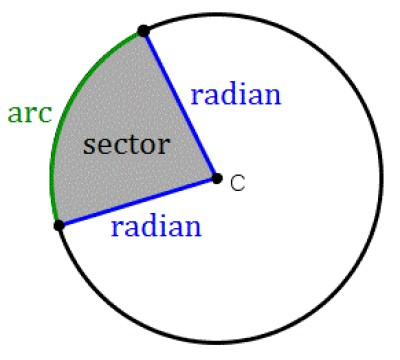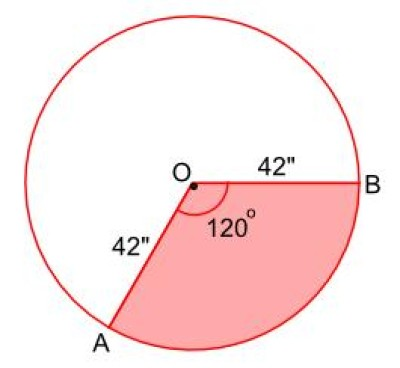# Length of an Arc

## Plain sticky notes

### Sticky note

Length arc = angle/360 x 2πr

### Sticky note

so... angle = 120 radii = 42" 120/360 = 1/3 42 x 2 = 80 = 0.33333 80 x π =251.33

## Photos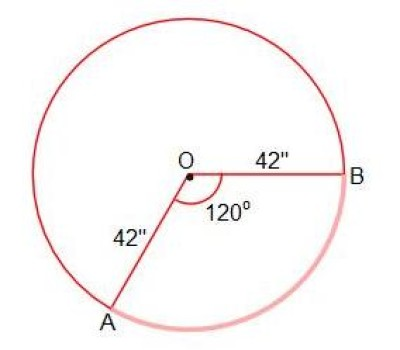# Area of Triangles

## Plain sticky notes

### 1/2 ab sin C

So side a is 10cm. side b is 8cm. and angle C is 50° the area will be 30.64

### (hxb) /2

So the height is 10cm and the base is 8cm. the area will be 40cm

So

## Photos

### Herons formula